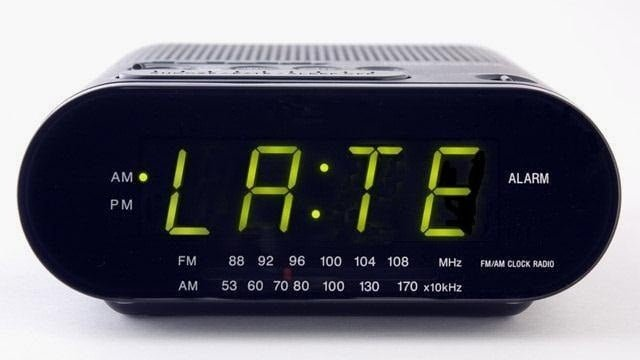# How to make 10 times profit in a day?You might be curious – Is it really possible? How can you make so much of profit in a day? 10 times profit – impossible !

So, Here how we do it.

## Step 1: Asses your risk less capital.

Your risk less capital is the minimum amount, which if you lose you don’t get affected financially. Suppose, you have INR 100,000 as total capital for trading purpose and you are comfortable with a loss of INR 2000 in a trade. This means INR 2000 is your risk less capital.

## Step 2: Wait till the expiry day of the option contract.

Choose a most liquid option contract. Here, I take NIFTY50 (index option).  Now, I’ll wait till the expiry day or a day before the expiry.

I’ll check ATR range using any technical analysis software for the day. You can use a free technical analysis tool at icharts.in

ATR stands for Average True Range. True range is the difference between High & Low of the day and 14 days average of true range can be taken.

Suppose, NIFTY Index is trading at 8285 and ATR for NIFTY50 is 120 points. This means on an average NIFTY can move +120 up or -120 down in a single trading day.

## Step 3: Choosing the right option contract

Here, things get crazy! Which option contract to choose? I’ll tell you simple approach to choose the right option contract.

1. Find out trend bullish, bearish or neutral !
2. If bullish, you need to buy an Out of the money Call option (In our Example NIFTY Index is at 8285. So, you can buy a 8300 Call. Premium on the expiry day will be around INR 2)
3. If bearish, you need to buy an out of the money put option (In our Example NIFTY Index is at 8285. So, you can buy a 8250 Put. Premium on the expiry day will be around INR 2)
4. If neutral market, buy an OTM call and buy OTM put (Buy 8300 Call and 8250 Put. Total premium will be INR 4 only)

## Step 4: Booking the profit / loss

In the above strategy your maximum loss will be INR 2000 (riskless capital). However, suppose your analysis about the market turns correct. You may have amazing returns.

Calculation:

NIFTY = 8275
Buy Call at = 8300 (Suppose, I’m bullish)
Investment: 2000
Total NIFTY: 2000/2 = 1000
Lot Size: 25
Total Lot: 1000/25= 40

Condition 1: If NIFTY goes Just 60 point up. (Half of the ATR)

Option Payoff = (8275+120)-8300 = 8335-8300 = 35
Profit on per NIFTY = 35-2= 33

Total Profit =  40 x 25 x 33 = 1000 x 33 = INR 33,000

Condition 2: If NIFTY goes Just 120 point up. (As per the ATR)

Option Payoff = (8275+120)-8300 = 8395-8300 = 95
Profit on per NIFTY = 95-2= 93

Total Profit =  40 x 25 x 93 = 1000 x 93 = INR 93,000

Condition 3: If NIFTY goes remains below 8300

Maximum loss of INR 2000

## Step 5: Precaution

This is a highly speculative strategy. So, profit completely depends on your analysis and accuracy of trend forecasting. If you are accurate in trend forecasting you’ll do awesome and if you need training in forecasting. Here, can join our Advance Technical Analysis course.

## Conclusion

Derivatives are great tool for risk management. However, you can equally use them for speculative strategies. It’s completely upto you. Please, keep in mind your risk taking capacity. Also, if you are highly interested in more Derivative strategies you can join our Derivative strategies program.

Don’t forget to write your comments in the box below and if you like the post share it with your facebook friends using social share buttons on the left of the screen.